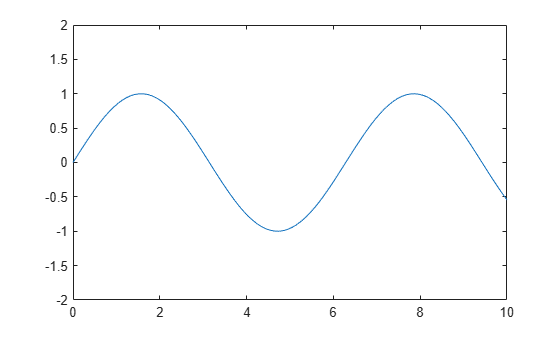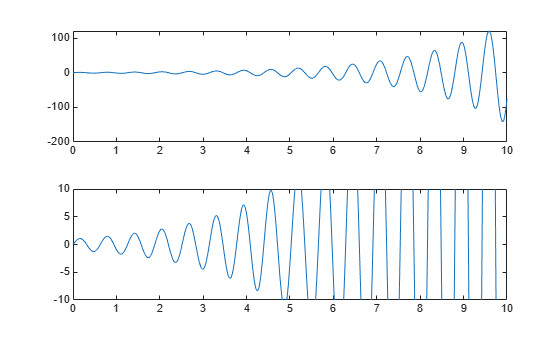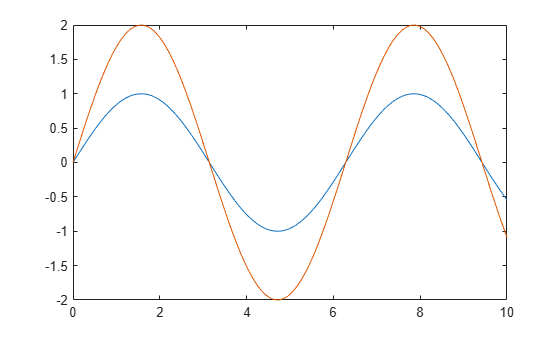# ylim

Set or query y-axis limits

## Syntax

``ylim(limits)``
``yl = ylim``
``ylim auto``
``ylim manual``
``m = ylim('mode')``
``___ = ylim(target,___)``

## Description

example

````ylim(limits)` sets the y-axis limits for the current axes or chart. Specify `limits` as a two-element vector of the form `[ymin ymax]`, where `ymax` is greater than `ymin`.```

example

````yl = ylim` returns the current limits as a two-element vector.```
````ylim auto` sets an automatic mode, enabling the axes to determine the y-axis limits. The limits span the range of the plotted data. Use this option if you change the limits and then want to set them back to the default values. This command sets the `YLimMode` property for the axes to `'auto'`.```

example

````ylim manual` sets a manual mode, freezing the limits at the current values. Use this option if you want to retain the current limits when adding new data to the axes using the `hold on` command. This command sets the `YLimMode` property for the axes to `'manual'`.```
````m = ylim('mode')` returns the current y-axis limits mode, which is either `'auto'` or `'manual'`. By default, the mode is automatic unless you specify limits or set the mode to manual.```

example

````___ = ylim(target,___)` uses the axes or chart specified by `target` instead of the current axes. Specify `target` as the first input argument for any of the previous syntaxes. You can include an output argument if the original syntax supports an output argument. Use single quotes around the mode inputs, for example, `ylim(target,'auto')`.```

## Examples

collapse all

Plot a line and set the y-axis limits to range from -2 to 2.

```x = linspace(0,10); y = sin(x); plot(x,y) ylim([-2 2])```Create a surface plot and show only y values greater than 0. Specify the minimum y-axis limit as 0 and let MATLAB choose the maximum limit.

```[X,Y,Z] = peaks; surf(X,Y,Z) ylim([0 inf])```Create a horizontal bar chart with dates along the y-axis. Set the y-axis limits to range from June 1, 2014 to June 10, 2014.

```t = datetime(2014,06,1) + caldays(0:20); y = rand(21,1); barh(t,y) tstart = datetime(2014,06,1); tend = datetime(2014,06,10); ylim([tstart tend])```Starting in R2019b, you can display a tiling of plots using the `tiledlayout` and `nexttile` functions. Call the `tiledlayout` function to create a 2-by-1 tiled chart layout. Call the `nexttile` function to create the axes objects `ax1` and `ax2`. Plot data into each axes. Then set the y-axis limits for the bottom plot by specifying `ax2` as the first input argument to `ylim`.

```tiledlayout(2,1) x = linspace(0,10,1000); y = sin(10*x).*exp(.5*x); ax1 = nexttile; plot(ax1,x,y) ax2 = nexttile; plot(ax2,x,y) ylim(ax2,[-10 10])```Use manual mode to maintain the current y-axis limits when you add more plots to the axes.

First, plot a line.

```x = linspace(0,10); y = sin(x); plot(x,y)```Set the y-axis limits mode to manual so that the limits to not change. Use `hold on` to add a second plot to the axes.

```ylim manual hold on y2 = 2*sin(x); plot(x,y2) hold off```The y-axis limits do not update to incorporate the new plot.

Switch back to automatically updated limits by resetting the mode to automatic.

`ylim auto`Create a scatter plot of random data. Return the values of the y-axis limits.

```x = randn(50,1); y = randn(50,1); scatter(x,y)````yl = ylim`
```yl = 1×2 -2 3 ```

## Input Arguments

collapse all

Minimum and maximum limits, specified as a two-element vector of the form `[ymin ymax]`, where `ymax` is greater than `ymin`. You can specify the limits as numeric, categorical, datetime, or duration values. However, the type of values that you specify must match the type of values along the y-axis.

You can specify both limits, or specify one limit and let MATLAB® automatically calculate the other. For an automatically calculated minimum or maximum limit, use `-inf` or `inf`, respectively.

Example: `ylim([0 1])`

Example: `ylim([-inf 1])`

Example: `ylim([0 inf])`

Data Types: `single` | `double` | `int8` | `int16` | `int32` | `int64` | `uint8` | `uint16` | `uint32` | `uint64` | `categorical` | `datetime` | `duration`

Target axes or chart, specified as an `Axes` object or a graphics object with an `YLimits` property, such as a `HeatmapChart` object. If you do not specify the axes or chart, then `ylim` sets the limits for the current axes or chart (returned by `gca`).

## Output Arguments

collapse all

Current limits, returned as a two-element vector of the form ```[ymin ymax]```.

Querying the limits returns the `YLim` or `YLimits` property value for the corresponding `Axes` or graphics object.

Current limits mode, returned as one of these values:

• `'auto'` — Automatically determine the limits.

• `'manual'` — Use manually specified limits that do not update to reflect changes in the data.

Querying the y-axis limits mode returns `YLimMode` property value for the corresponding `Axes` object.

## Algorithms

The `ylim` function sets and queries several axes properties related to the y-axis limits.

• `YLim` — Property that stores the y-axis limits.

• `YLimMode` — Property that stores the y-axis limits mode. When you set the y-axis limits, this property changes to `'manual'`.